# Research

## 2022

#### Manifold learning

Elodie Maignant, Xavier Pennec, Alain Trouvé

In this work, we review popular manifold learning methods relying on the notion of neighbour graph (Laplacian Eigenmaps, Isomap, LLE, t-SNE) with a particular focus on Locally Linear Embedding (LLE). This includes:
– A deeper analysis of the different methods [hal-03909427].
– Comparative studies on symptomatic and shape analysis examples.
– The generalisation of the methods to manifold-valued data.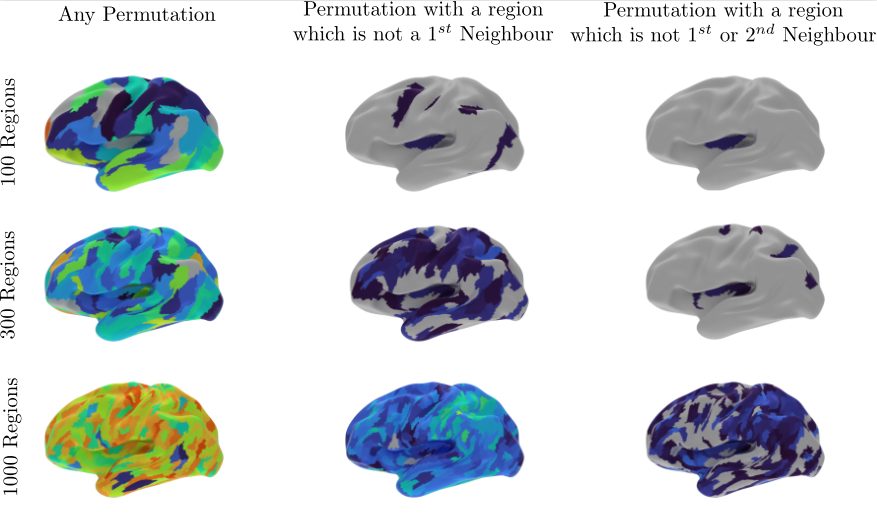Surface visualization of the permutation type. For each regions, we considered the rate at which the optimal graph matching permutes a regions with any other region (i.e. not a self permutation) [left column], with a region that is not a 1st degree neighbour [middle column], and with a region that is not a 1st or 2nd degree neighbour [right column]. Warmer and colder colours indicate a higher and lower permutation rates, respectively. Gray indicates no permutation. The three rows correspond to a brain parcellation with 100, 300, and 1000 regions. Our results suggest that the optimal graph alignment of regions should be performed before further analysis but optimal permutations are in the great majority local within 1st or second degree neighbours, which drastically simplifies the algorithmic search.

#### Graph Alignment Exploiting the Spatial Organisation Improves the Similarity of Brain Networks

Anna Calissano, Samuel Deslauriers-Gauthier, Theodore Papadopoulo, Xavier Pennec.

Can the structural and functional properties of a specific brain region of an atlas be assumed to be the same across subjects? In [hal-03910761] we addressed this question by looking at the network representation of the brain, with nodes corresponding to brain regions and edges to their structural relationships. We perform graph matching on a set of control patients and on parcellations of different granularity to measure the connectivity misalignment between regions. The graph matching is unsupervised and reveals interesting insight on local misalignment of brain regions
across subjects as shown in the figure.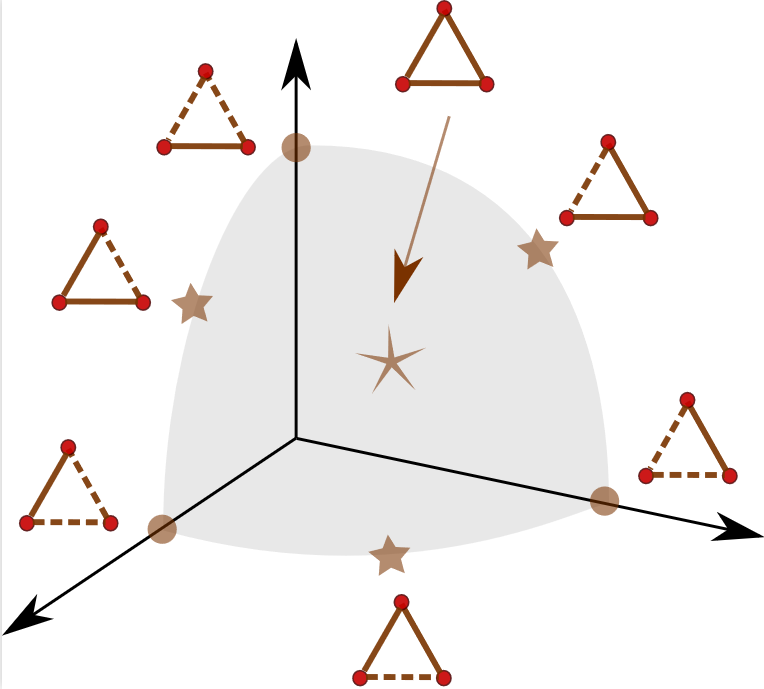Visualization of the Currents Space of Graphs for $K_3$.

#### The Currents Space of Graphs

James Benn, Anna Calissano, Stephen Marsland, Xavier Pennec.

We defined in [hal-03910825] a novel embedding space for binary undirected graphs, the Currents Spaces of Graphs. We represent simple graphs on n vertices by 1-forms over a complete graph K_n. It is shown that these 1-forms lie on a hypersphere in the Hilbert space L^2 (\Omega^1_{K_n}) of all 1-forms and the round metric induces a natural distance metric on graphs. The metric itself reduces to a global spherical Jaccard-type metric which can be computed from edge-list data alone. The structure of the graph space embedding for three vertices is illustrated in the figure.
Lastly, we describe the global structure of the 1-forms representing graphs and how these can be exploited for the statistical analysis of a sample of graphs.

#### The Measurement and Analysis of Shape: An Application of hydrodynamics and probability theory

James Benn, Stephen Marsland.

A de Rham p-current can be viewed as a map (the current map) between the set of embeddings of a closed p-dimensional manifold into an ambient n-manifold and the set of linear functionals on differential p-forms. We demonstrate that, for suitably chosen Sobolev topologies on both the space of embeddings and the space of p-forms, the current map is continuously differentiable, with an image that consists of bounded linear functionals on p-forms. Using the Riesz representation theorem we prove that each p-current can be represented by a unique co-exact differential form that has a particular interpretation depending on p.
Embeddings of a manifold can be thought of as shapes with a prescribed topology. Our analysis of the current map provides us with representations of shapes that can be used for the measurement and statistical analysis of collections of shapes. We consider two special cases of our general analysis and prove that: (1) if p=n-1 then closed, embedded, co-dimension one surfaces are naturally represented by probability distributions on the ambient manifold; and (2) if p=1 then closed, embedded, one-dimensional curves are naturally represented by fluid flows on the ambient manifold. In each case we outline some statistical applications using an \dot{H}^{1} and L^{2} metric, respectively. [hal-03556752]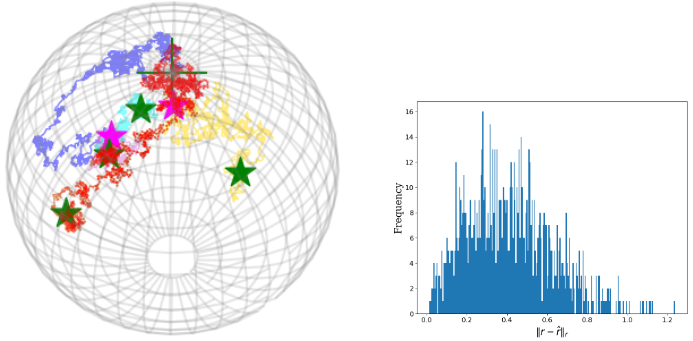Left: A realization of a phylogenetic tree generated from Brownian motions on the sphere. The green ‘+’ is the root node. The 4 green stars are leaf nodes. The two pink stars are inner nodes. Right: Histogram of the geodesic distance between the true root r and the estimated root \hat{r}, for 1000 simulated trees on the sphere. For reference, the geodesic distance from the north pole (the true root) to a point on the equator is \pi/2 \approx 1.57. The histogram shows that the error made by our method in estimating the root-node is low and well behaved in this setting.

#### Tangent phylogenetic PCA

Morten Pedersen, Stefan Sommer, Xavier Pennec.

Phylogenetic PCA (p-PCA) is a well-known version of PCA for observations that are leaf nodes of a phylogenetic tree. The method works on Euclidean data, but in evolutionary biology there is a need for applying it to data on manifolds, particularly shape-trees. We provide in [hal-03842847] a generalization of p-PCA to data lying on Riemannian manifolds, called Tangent p-PCA. The figure illustrates step 1 of our method, consisting of estimating the unknown root node of the phylogenetic tree.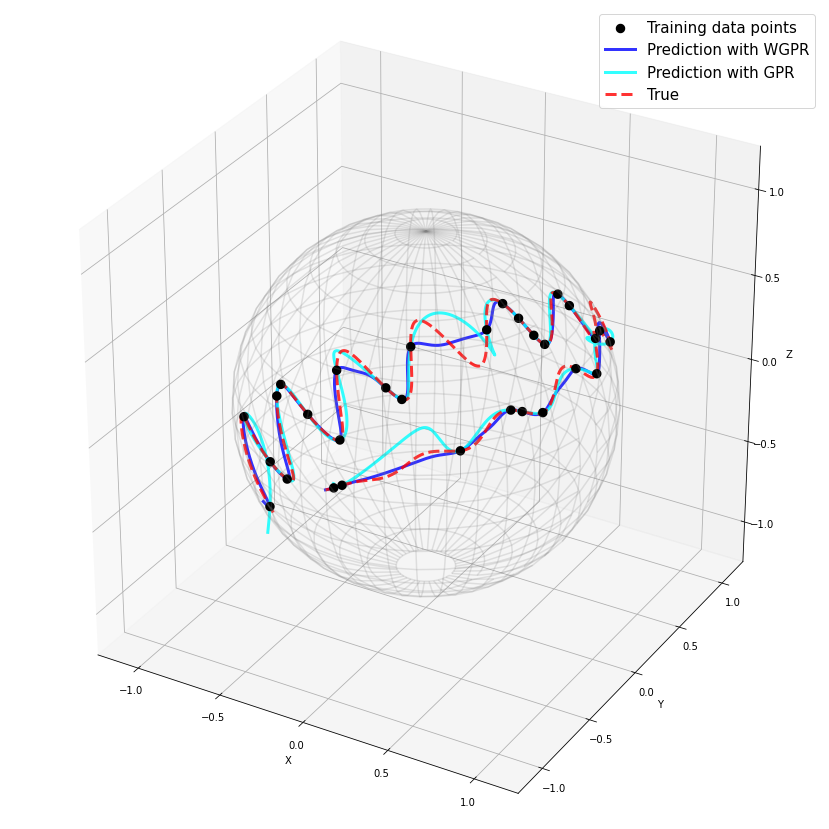Sphere dataset (black points) interpolated using Gaussian Process Regression (cyan) and Wrapped Gaussian Process Regression (blue).

#### Wrapped Gaussian Process Regression on Riemannian Manifolds

Tom Szwagier, Arthur Pignet.

This work focuses on the implementation in the python library Geomstats of wrapped Gaussian process regression on Riemannian manifolds. This work won the second prize from the ICLR Computational Geometry &Topology Challenge associated to the ICLR 2022 Workshop on Geometrical and Topological Representation Learning. The challenge and its submissions are summarized in the white paper [hal-03903044] and the code is available on the challenge repository.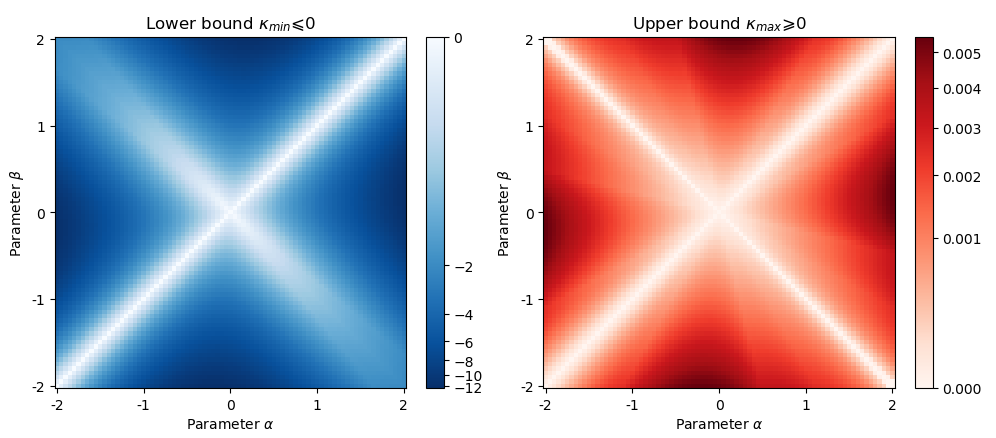Lower and upper bounds of the sectional curvature of the Mixed-Power-Euclidean metrics.

#### Geometries of covariance and correlation matrices

Yann Thanwerdas, Xavier Pennec.

In [hal-03414887], we use the principles of deformed metrics and balanced metrics to:
1. introduce Mixed-Power-Euclidean metrics which encompass the Euclidean, affine-invariant, log-Euclidean and BKM metrics;
2. relate the MPE metrics to the (u,v)-divergences of Information Geometry;
3. compute the curvature (see bounds on the figure).

In [tel-03698752], we further:
1. introduce convenient metrics on correlation matrices of full rank;
2. characterize the Bures-Wasserstein geodesics on covariance matrices.